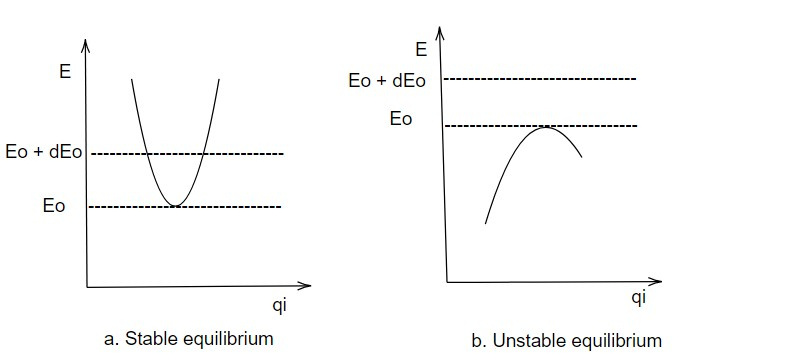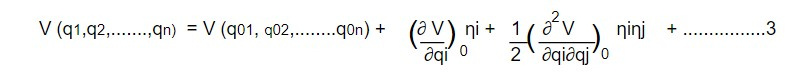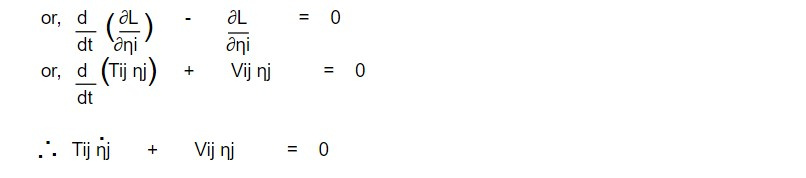# Small oscillations

Lagrangian approach is suitable for the system with small oscillation about an equilibrium position. The theory of small oscillation about equilibrium position is applicable in molecular spectra, vibrations of mechanics and coupled electrical circuits.

Lagrangian equation of motion for small oscillations:

Let us consider a conservative system in which the potential energy is a function of position only. Again, we take such a system in which the transformation equations of generalized coordinates q1,q2,.....qn do not involve time explicitly. Thus, time dependent constants are to be excluded. The system is said to be in equilibrium when the generalized force acting on the system vanish. i.e.

Qi = -( ∂V/ ∂qi ) = 0....1

If a system is in equilibrium with zero initial velocity, then it will continue in its state of equilibrium for infinitely long time. At equilibrium potential energy is extremum (either maximum or minimum). Example of equilibrium mechanical system are

i. Pendulum at rest

ii. An egg standing on one end

There are two kinds of equilibrium: One is stable and another is unstable. An equilibrium is said to be stable, small disturbance from its equilibrium position results only in small bounded motion about the mean position. And an equilibrium position results in unbounded motion. A pendulum at rest is in stable equilibrium but an egg standing on and is an unstable equilibrium.

At equilibrium, potential energy can either maximum or minimum. If potential energy is minimum at equilibrium then any increase in the energy of the system we increase the potential energy (V) and subsequently these will be decrease in kinetic energy (T) so that system will immediately return to the equilibrium position i.e a system at equilibrium with minimum potential energy is at stable equilibrium. On the other hand if V decreases at a result of some departure(leaving a place at a particular time) from the equilibrium, the kinetic and velocity increase indefinitely corresponding to unstable motion.

The stable and unstable equilibrium can be shown below:We are interested for the motion of the system in the neighbourhood of stable equilibrium. Let qoi denote the generalized coordinate system at equilibrium and ηi be the small deviation of the generalised coordinate system from equilibrium position. Then the generalised coordinate qi can be expressed as

qi = qoi + ηi

V(qi ) = V(qoi + ηi)........2

Expanding the potential energy about qoi , we obtainThe second term of equation 3 vanishes due to equation 1 and the first term in the series is the potential energy of equilibrium position and by shifting the arbitrary zero of potential to coincide with the equilibrium potential, this term may be made to vanish then equation 3 becomesLet us represent the derivative of V by Vij and Vij is symmetric i.e Vij = Vji then equation 4 becomesSimilar treatment for kinetic energy iswhere Tij = Tji i.e Tij is also symmetric.

Now Lagrangian can be obtained as

L = T- VTaking η's as generalised coordinates, the Lagrangian of equation 7 gives the following n equations of motion,which is the required equation of motion of Lagrangian for small oscillation.

This note is taken from Classical Mechanics, MSC physics, Nepal.

This note is a part of the Physics Repository.